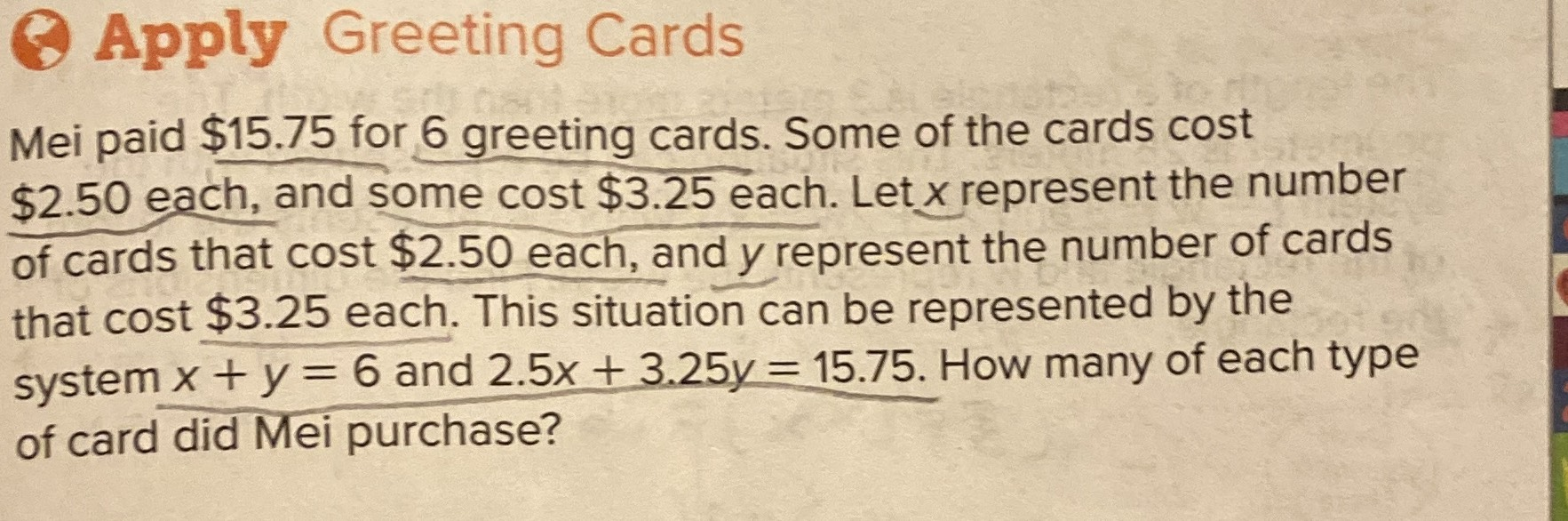### ¿Todavía tienes preguntas de matemáticas?

Pregunte a nuestros tutores expertos
Algebra
Pregunta(8pply Greeting Cards Mei paid $$\ 15.75$$ for $$6$$ greeting cards. Some of the cards cost $$\ 2.50$$ each, and some cost $$\ 3.25$$ each. Let $$x$$ represent the number of cards that cost $$\ 2.50$$ each, and $$y$$ represent the number of cards that cost $$\ 3.25$$ each. This situation can be represented by the system $$x + y = 6$$ and $$2.5 x + 3.25 y = 15.75$$ . How many of each type of card did Mei purchase?